• 实现矩阵压缩存储，并实现快速转置，大大减少了转置的时间复杂度。
• 实验报告 课程名数据结构 C 语言版 实验名矩阵压缩存储 姓 名 班 级 学 号 时 间2014.11.23 一 实验目的与要求 1. 掌握并实现稀疏矩阵压缩存储的方法 2. 在该存储方法上实现矩阵的操作 二 实验内容 ? 判断一个用...
• 1.测试对称矩阵压缩 test1文件的内容： 界面输入如下： 下拉组合选择框，选择对称矩阵，点击按钮“压缩”。 运行结果：输出文件1.1.txt文件内容如下： 上三角矩阵、下三角矩阵、稀疏矩阵的测试方式同上。 代码 ...
运行界面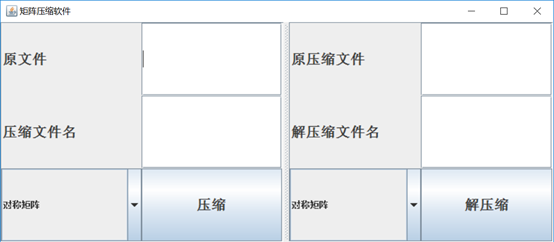PS:测试时需要自己在目录C:\Users\win10\Desktop 下先建立测试文件。
一、
1.测试对称矩阵的压缩
test1文件的内容：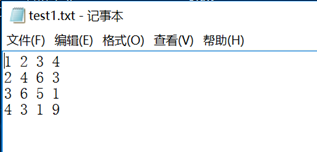界面输入如下：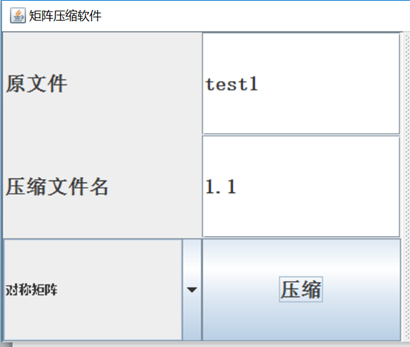下拉组合选择框，选择对称矩阵，点击按钮“压缩”。
运行结果：输出文件1.1.txt文件内容如下：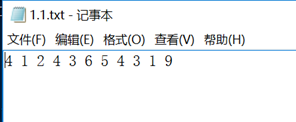上三角矩阵、下三角矩阵、稀疏矩阵的测试方式同上。
代码
//画界面需要的
import java.awt.Font;
import java.awt.GridLayout;
import java.awt.Label;
import java.awt.event.ActionEvent;
import java.awt.event.ActionListener;
import java.io.BufferedWriter;
//文件读写需要的
import java.io.File;
import java.io.FileInputStream;
import java.io.FileNotFoundException;
import java.io.FileOutputStream;
import java.io.IOException;
import java.io.OutputStreamWriter;
import java.io.UnsupportedEncodingException;
import java.util.Iterator;

import javax.swing.*;

import javax.swing.JButton;
import javax.swing.JFrame;
import javax.swing.JPanel;
import javax.swing.JSplitPane;
import javax.swing.JTextField;

public class yasuo extends JFrame implements ActionListener{

/**
*
*/
private static final long serialVersionUID = 4088474481798858796L;
protected JComboBox<String> juzhentype1;//组合框
protected JComboBox<String> juzhentype2;//组合框
private JTextField yuan1field;
private JTextField yafield;
private JTextField yuan2field;
private JTextField jieyafield;

private JButton yabutton;
private JButton jiebutton;

private JPanel leftpanel;   //面板流布局
private JPanel rightpanel;

public yasuo()
{
super("矩阵压缩软件");
this.setBounds(500, 400, 800, 350);
leftpanel=new JPanel();
rightpanel=new JPanel();

JSplitPane split = new JSplitPane(JSplitPane.HORIZONTAL_SPLIT, leftpanel, rightpanel);
split.setDividerLocation(400);                     //设置水平分隔条的位置

yabutton=new JButton("压缩");
yabutton.setFont(new Font("宋体",Font.BOLD,20));
jiebutton=new JButton("解压缩");
jiebutton.setFont(new Font("宋体",Font.BOLD,20));

yuan1field= new JTextField(6);
yuan1field.setHorizontalAlignment(JTextField.LEFT); //设置向左对齐
yuan1field.setFont(new Font("宋体",Font.BOLD,20));  //设置字体格式
Label label1=new Label("原文件",Label.LEFT);
label1.setFont(new Font("宋体",Font.BOLD,20));

yafield= new JTextField(8);
yafield.setHorizontalAlignment(JTextField.LEFT); //设置向左对齐
yafield.setFont(new Font("宋体",Font.BOLD,20));  //设置字体格式
Label label2=new Label("压缩文件名",Label.LEFT);
label2.setFont(new Font("宋体",Font.BOLD,20));

String type[]= {"对称矩阵","稀疏矩阵","上三角矩阵","下三角矩阵"};
juzhentype1=new JComboBox<String>(type);
juzhentype2=new JComboBox<String>(type);

leftpanel.setLayout(new GridLayout(3,3));

yuan2field= new JTextField(6);
yuan2field.setHorizontalAlignment(JTextField.LEFT); //设置向左对齐
yuan2field.setFont(new Font("宋体",Font.BOLD,20));  //设置字体格式
Label label3=new Label("原压缩文件",Label.LEFT);
label3.setFont(new Font("宋体",Font.BOLD,20));

jieyafield= new JTextField(8);
jieyafield.setHorizontalAlignment(JTextField.LEFT); //设置向左对齐
jieyafield.setFont(new Font("宋体",Font.BOLD,20));  //设置字体格式
Label label4=new Label("解压缩文件名",Label.LEFT);
label4.setFont(new Font("宋体",Font.BOLD,20));

rightpanel.setLayout(new GridLayout(3,3));

//this.setBackground(java.awt.Color.lightGray);
this.setDefaultCloseOperation(EXIT_ON_CLOSE);
this.setVisible(true);
}

public void actionPerformed(ActionEvent ev) {

//压缩
if(ev.getSource()==yabutton)
{
if(juzhentype1.getSelectedIndex()==0) //对称矩阵
{
System.out.println("压缩");
String yuan1=(yuan1field.getText());
String ya=(yafield.getText());

//新建文件夹
File fileout = new File("C:\\Users\\win10\\Desktop", ya+".txt");
try
{
fileout.createNewFile(); // 创建文件
}
catch (IOException e)
{
e.printStackTrace();
}

String fileinpath="C:/Users/win10/Desktop/"+yuan1+".txt";
File filein = new File(fileinpath);

OutputStreamWriter outStream = null;
try {
"UTF-8");
outStream = new OutputStreamWriter(new FileOutputStream(fileout),
"UTF-8");
} catch (UnsupportedEncodingException e) {
e.printStackTrace();
} catch (FileNotFoundException e) {
e.printStackTrace();
}

BufferedWriter writer = new BufferedWriter(outStream);

String result;
String numarray[];
int n=0;
try {
numarray = result.split(" ");
n=numarray.length;
writer.write(String.valueOf(n));
writer.write(" ");
writer.write(numarray);
writer.write(" ");
int i=2;
{
numarray = result.split(" ");

for(int j=0;j<i;j++)
{
writer.write(numarray[j]);
writer.write(" ");
}
i++;
}
writer.flush();
writer.close();
} catch (IOException e) {
e.printStackTrace();
} finally {
try {
}
if (writer != null) {
writer.close();
}
} catch (IOException e) {
e.printStackTrace();
}
}

}
else if(juzhentype1.getSelectedIndex()==1) //稀疏矩阵
{
System.out.println("压缩");
String yuan1=(yuan1field.getText());
String ya=(yafield.getText());

//新建文件夹
File fileout = new File("C:\\Users\\win10\\Desktop", ya+".txt");
try
{
fileout.createNewFile(); // 创建文件
}
catch (IOException e)
{
e.printStackTrace();
}

String fileinpath="C:/Users/win10/Desktop/"+yuan1+".txt";
File filein = new File(fileinpath);

OutputStreamWriter outStream = null;
try {
"UTF-8");
outStream = new OutputStreamWriter(new FileOutputStream(fileout),
"UTF-8");
} catch (UnsupportedEncodingException e) {
e.printStackTrace();
} catch (FileNotFoundException e) {
e.printStackTrace();
}

BufferedWriter writer = new BufferedWriter(outStream);

String result;
String numarray[];

int k=0;
int n=0,m=0;//n:行，m：列
try {
{
n++;
}
numarray = juz.getFirst().split(" ");
m=numarray.length;
writer.write(String.valueOf(n));
writer.write(" ");
writer.write(String.valueOf(m));
writer.newLine();
Iterator<String> juzz=juz.descendingIterator();
for(int i=n;i>0;i--)
{
for(int j=0;j<m;j++)
{
if(!numarray[j].equals("0"))
{
writer.write(String.valueOf(i));
writer.write(" ");
writer.write(String.valueOf(j));
writer.write(" ");
writer.write(numarray[j]);
writer.newLine();
}
}
numarray = juzz.next().split(" ");

}
writer.flush();
writer.close();
} catch (IOException e) {
e.printStackTrace();
} finally {
try {
}
if (writer != null) {
writer.close();
}
} catch (IOException e) {
e.printStackTrace();
}
}
}
else if(juzhentype1.getSelectedIndex()==2) //上三角矩阵
{
System.out.println("压缩");
String yuan1=(yuan1field.getText());
String ya=(yafield.getText());

//新建文件夹
File fileout = new File("C:\\Users\\win10\\Desktop", ya+".txt");
try
{
fileout.createNewFile(); // 创建文件
}
catch (IOException e)
{
e.printStackTrace();
}

String fileinpath="C:/Users/win10/Desktop/"+yuan1+".txt";
File filein = new File(fileinpath);

OutputStreamWriter outStream = null;
try {
"UTF-8");
outStream = new OutputStreamWriter(new FileOutputStream(fileout),
"UTF-8");
} catch (UnsupportedEncodingException e) {
e.printStackTrace();
} catch (FileNotFoundException e) {
e.printStackTrace();
}

BufferedWriter writer = new BufferedWriter(outStream);

String result;
String numarray[];
int n=0;
try {
numarray = result.split(" ");
n=numarray.length;
writer.write(String.valueOf(n));
writer.write(" ");
writer.write(result);
writer.write(" ");
int i=1;
{
numarray = result.split(" ");

for(int j=i;j<n;j++)
{
writer.write(numarray[j]);
writer.write(" ");
}
i++;
}
writer.flush();
writer.close();
} catch (IOException e) {
e.printStackTrace();
} finally {
try {
}
if (writer != null) {
writer.close();
}
} catch (IOException e) {
e.printStackTrace();
}
}
}
else if(juzhentype1.getSelectedIndex()==3) //下三角矩阵
{
System.out.println("压缩");
String yuan1=(yuan1field.getText());
String ya=(yafield.getText());

//新建文件夹
File fileout = new File("C:\\Users\\win10\\Desktop", ya+".txt");
try
{
fileout.createNewFile(); // 创建文件
}
catch (IOException e)
{
e.printStackTrace();
}

String fileinpath="C:/Users/win10/Desktop/"+yuan1+".txt";
File filein = new File(fileinpath);

OutputStreamWriter outStream = null;
try {
"UTF-8");
outStream = new OutputStreamWriter(new FileOutputStream(fileout),
"UTF-8");
} catch (UnsupportedEncodingException e) {
e.printStackTrace();
} catch (FileNotFoundException e) {
e.printStackTrace();
}

BufferedWriter writer = new BufferedWriter(outStream);

String result;
String numarray[];
int n=0;
try {
numarray = result.split(" ");
n=numarray.length;
writer.write(String.valueOf(n));
writer.write(" ");
writer.write(numarray);
writer.write(" ");
int i=2;
{
numarray = result.split(" ");

for(int j=0;j<i;j++)
{
writer.write(numarray[j]);
writer.write(" ");
}
i++;
}
writer.flush();
writer.close();
} catch (IOException e) {
e.printStackTrace();
} finally {
try {
}
if (writer != null) {
writer.close();
}
} catch (IOException e) {
e.printStackTrace();
}
}
}

}

//解压缩
else if(ev.getSource()==jiebutton)
{
if(juzhentype2.getSelectedIndex()==0)  //对称矩阵
{
System.out.println("解压缩");
String yuan2=(yuan2field.getText());
String jieya=(jieyafield.getText());

//新建文件夹
File fileout = new File("C:\\Users\\win10\\Desktop", jieya+".txt");
try
{
fileout.createNewFile(); // 创建文件
}
catch (IOException e)
{
e.printStackTrace();
}

String fileinpath="C:/Users/win10/Desktop/"+yuan2+".txt";
File filein = new File(fileinpath);

OutputStreamWriter outStream = null;
try {
"UTF-8");
outStream = new OutputStreamWriter(new FileOutputStream(fileout),
"UTF-8");
} catch (UnsupportedEncodingException e) {
e.printStackTrace();
} catch (FileNotFoundException e) {
e.printStackTrace();
}

BufferedWriter writer = new BufferedWriter(outStream);

String result;
String numarray[];
int n=0;
try {
numarray = result.split(" "); //numarray存储的第一行居然是个空格。

//writer.write(numarray);
for(int i=1;i<=n;i++)
{
for(int j=1;j<=n;j++)
{
if(i<j)
{
writer.write(numarray[j*(j-1)/2+i-1]);
writer.write(" ");
}
else if(i>=j)
{
writer.write(numarray[i*(i-1)/2+j-1]);
writer.write(" ");
}
}

writer.newLine();
}
writer.flush();
}
writer.close();
} catch (IOException e) {
e.printStackTrace();
} finally {
try {
}
if (writer != null) {
writer.close();
}
} catch (IOException e) {
e.printStackTrace();
}
}

}
else if(juzhentype2.getSelectedIndex()==1) //稀疏矩阵
{
System.out.println("解压缩");
String yuan2=(yuan2field.getText());
String jieya=(jieyafield.getText());

//新建文件夹
File fileout = new File("C:\\Users\\win10\\Desktop", jieya+".txt");
try
{
fileout.createNewFile(); // 创建文件
}
catch (IOException e)
{
e.printStackTrace();
}

String fileinpath="C:/Users/win10/Desktop/"+yuan2+".txt";
File filein = new File(fileinpath);
OutputStreamWriter outStream = null;
try {
"UTF-8");
outStream = new OutputStreamWriter(new FileOutputStream(fileout),
"UTF-8");//GBK
} catch (UnsupportedEncodingException e) {
e.printStackTrace();
} catch (FileNotFoundException e) {
e.printStackTrace();
}

BufferedWriter writer = new BufferedWriter(outStream);

String result;

int n=0,m=0;
try {
//System.out.println(n+","+m);
int[][] numarray;
numarray=new int[];
String[][] array=new String[n][m];
for(int x=0;x<n;x++)
{
for(int y=0;y<m;y++)
{
array[x][y]="0";
}
}

{

String resultt[]=result.split(" ");
int a=Integer.parseInt(resultt);
int b=Integer.parseInt(resultt);
String c=resultt;
array[a][b]=c;

}

for(int a=0;a<n;a++)
{
for(int b=0;b<m;b++)
{
writer.write(array[a][b]);
writer.write(" ");
}
writer.newLine();
}

writer.flush();
writer.close();
} catch (IOException e) {
e.printStackTrace();
} finally {
try {
}
if (writer != null) {
writer.close();
}
} catch (IOException e) {
e.printStackTrace();
}
}

}
else if(juzhentype2.getSelectedIndex()==2) //上三角矩阵
{
System.out.println("解压缩");
String yuan2=(yuan2field.getText());
String jieya=(jieyafield.getText());

//新建文件夹
File fileout = new File("C:\\Users\\win10\\Desktop", jieya+".txt");
try
{
fileout.createNewFile(); // 创建文件
}
catch (IOException e)
{
e.printStackTrace();
}

String fileinpath="C:/Users/win10/Desktop/"+yuan2+".txt";
File filein = new File(fileinpath);
// File fileOut = new File("C:/Users/win10/Desktop/test2.txt");

OutputStreamWriter outStream = null;
try {
"UTF-8");
outStream = new OutputStreamWriter(new FileOutputStream(fileout),
"UTF-8");
} catch (UnsupportedEncodingException e) {
e.printStackTrace();
} catch (FileNotFoundException e) {
e.printStackTrace();
}

BufferedWriter writer = new BufferedWriter(outStream);

String result;
String numarray[];
int n=0;
try {
numarray = result.split(" "); //numarray存储的第一行居然是个空格。
int k=0; //记录numarray的下标。

//writer.write(numarray);
for(int i=0;i<n;i++)
{
int j=0;
while(j<i)
{
writer.write("0");
writer.write(" ");
j++;
}
while(i<=j&&j<n)
{
writer.write(numarray[k]);
writer.write(" ");
k++;j++;
}

writer.newLine();
}
writer.flush();
}
writer.close();
} catch (IOException e) {
e.printStackTrace();
} finally {
try {
}
if (writer != null) {
writer.close();
}
} catch (IOException e) {
e.printStackTrace();
}
}

}

else if(juzhentype2.getSelectedIndex()==3)  //下三角矩阵
{
System.out.println("解压缩");
String yuan2=(yuan2field.getText());
String jieya=(jieyafield.getText());

//新建文件夹
File fileout = new File("C:\\Users\\win10\\Desktop", jieya+".txt");
try
{
fileout.createNewFile(); // 创建文件
}
catch (IOException e)
{
e.printStackTrace();
}

String fileinpath="C:/Users/win10/Desktop/"+yuan2+".txt";
File filein = new File(fileinpath);

OutputStreamWriter outStream = null;
try {
"UTF-8");
outStream = new OutputStreamWriter(new FileOutputStream(fileout),
"UTF-8");
} catch (UnsupportedEncodingException e) {
e.printStackTrace();
} catch (FileNotFoundException e) {
e.printStackTrace();
}

BufferedWriter writer = new BufferedWriter(outStream);

String result;
String numarray[];
int n=0;
try {
numarray = result.split(" "); //numarray存储的第一行居然是个空格。
int k=0; //记录numarray的下标。

//writer.write(numarray);
for(int i=0;i<n;i++)
{
int j=0;
while(j<=i)
{
writer.write(numarray[k]);
writer.write(" ");
k++;j++;
}
while(j>i&&j<n)
{
writer.write("0");
writer.write(" ");
j++;
}

writer.newLine();
}
writer.flush();
}
writer.close();
} catch (IOException e) {
e.printStackTrace();
} finally {
try {
}
if (writer != null) {
writer.close();
}
} catch (IOException e) {
e.printStackTrace();
}
}

}
}

}

public static void main(String[] args)
{
new yasuo();
}

}


.思路总结
整体战略
先设计这个UI界面，考虑哪些内容是需要交互的。 我把整个界面一分为二，分别对应两个功能：压缩和解压缩。 每个功能都需要分别对四种类型的矩阵对象进行操作，因此考虑使用组合框以供用户选择压缩类型，这是第一层交互。 压缩对象（即具体处理那个文件）需要用户自由指定，操作完成后生成的文件名也需要用户指定，因此考虑用文本框以供输入文字，这是第二层交互。 界面设计完成，整体的框架也就可以了。剩下的只需要根据用户选择的功能种类和矩阵类型分成八大块，具体在对动作事件的响应里实现就好啦。 2. 重点对象
四种压缩矩阵里，其实可以只当成两种，即稀疏矩阵和对称矩阵。 稀疏矩阵，考虑用三元组进行存储。 上三角矩阵，下三角矩阵可以当成对称矩阵的简化版，这里重点考虑对对称矩阵的操作。 解压缩算法的核心在于：找到二维矩阵中元素下标 （i , j）与一维数组元素下标 k 之间的对应关系。自拟定不同的已知条件就有了不同时间复杂度的算法：
第一种：时间复杂度 O(n^2)

自拟定 i , j 都是已知的。 （解释一下我这里所谓的自拟定的含义：比如一层循环的遍历，其中的渐变量为x，那么我就叫x是自拟定已知的。因为对于x的遍历，对于循环中的每一次来说，x的值是已知的。也就是说，在我的理解里：对于某一个数的循环遍历，就是把这个原本是变量（有许多取值）的数，拆解为多个可能的常量，循环中的任意一次都是对其中某个可能常量的尝试） 即由k 去对应（i ， j）时，分别由 i ，j 组成二重循环遍历，去尝试i , j的值，因此时间复杂度是n的平方。
第二种：时间复杂度O(n) 自拟定i 为已知的。 由于：k=1/2*(2n – i)(i – i) + j 证明如下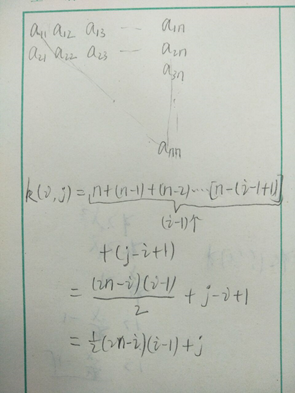所以：j = k - 1/2*(2n – i)(i – 1) 即由k 去对应（i ， j）时，由 i 组成一重循环遍历，去尝试i 的值，计算 j 的值，因此时间复杂度是n。
第三种：时间复杂度O(1)
j = k - 1/2*(2n – i)(i – i)
i=∟(n+3/2) - √n^2+n-2*k+9/4 」
即由k 去对应（i ， j）时，直接计算 i，j 值。
公式证明如下：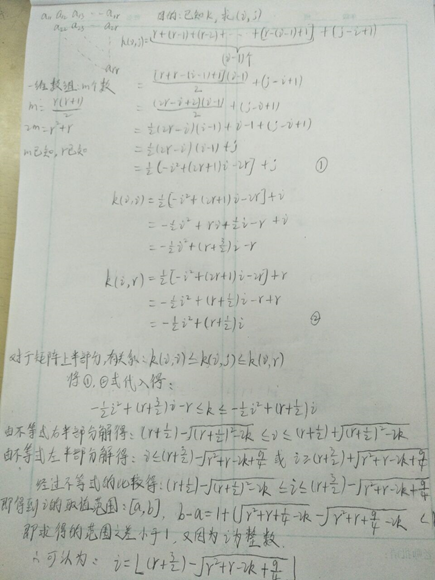展开全文java 数据结构
• 数据结构 禅设计报告 题 目 专 业 班 级 学 号 姓 名 指导老师 时 间: _课程设计题目及所涉及知识点 设计题目是矩阵的运算所涉及的知识点主要是 1 数据结构中的对于结构体的定义 用typedef struct来实现根据所设计的...
• 矩阵压缩存储之稀疏矩阵详解（C语言版）


文章目录
一、定义二、结构三、常用操作结语附录

一、定义
矩阵中非零元素的个数远远小于矩阵元素的总数，并且非零元素的分布没有规律，通常认为矩阵中非零元素的总数比上矩阵所有元素总数的值小于等于0.05时，则称该矩阵为稀疏矩阵(sparse matrix)。
二、结构
如何进行稀疏矩阵的压缩存储呢?         按照压缩存储的概念，只存储稀疏矩阵的非零元。因此，除了存储非零元的值之外，还必须同时记下它所在行和列的位置( i，j )。反之，一个三元组(i，j，e)惟一确定了矩阵A的一个非零元。由此，稀疏矩阵可由表示非零元的三元组及其行列数惟一确定。
#define ElemType int //矩阵内的数据类型
#define MAXSIZE 100   //矩阵内最大元素个数

//三元组结构
typedef struct Triple
{
int i;  //元素所在行
int j;  //元素所在列
ElemType e;  //元素值
}Triple;

//稀疏矩阵
typedef struct SMatrix
{
Triple data[MAXSIZE]; // 0 三元组，存放有效数据
int mu; //稀疏矩阵行数
int nu; //稀疏矩阵列数
int tu; //非零元素个数
}SMatrix;

三、常用操作
1. 创建稀疏矩阵
通过文件创建稀疏矩阵
//通过文件创建稀疏矩阵
void CreateMatrixByFile(SMatrix *M)
{
//创建文件指针，来读取存放在Matrix.txt中的矩阵信息
FILE *fp = fopen("Matrix.txt","r");
//判断文件是否存在
if(fp == NULL)
exit(1);
//读数据：矩阵的行mu，矩阵的列nu
fscanf(fp,"%d %d",&M->mu, &M->nu);

//读取矩阵内的数据
int value;  //临时存放读取的值，用来判断读取的值是否为0
int k = 0; //记录非零元素个数
for(int i=0; i<M->mu; ++i)
{
for(int j=0; j<M->nu; ++j)
{
//读取矩阵内的一个元素
fscanf(fp,"%d",&value);
if(value != 0)//判断值是不是为0
{//不为0，将值以三元组的形式存入
M->data[k].e = value; //值
M->data[k].i = i;   //所在行
M->data[k].j = j;   //所在列
k++; //非零元素个数加一
}
}
}
M->tu = k; //更改稀疏矩阵中非零元素的个数

//关闭文件指针
fclose(fp);
}


通过输入创建稀疏矩阵
//通过输入创建稀疏矩阵
void CreateMatrixByScanf(SMatrix *M)
{

//读数据：矩阵的行mu，矩阵的列nu
scanf("%d%d",&M->mu, &M->nu);

//读取矩阵内的数据
int value;  //临时存放读取的值，用来判断读取的值是否为0
int k = 0; //记录非零元素个数
for(int i=0; i<M->mu; ++i)
{
for(int j=0; j<M->nu; ++j)
{
//读取矩阵内的一个元素
scanf("%d",&value);
if(value != 0)//判断值是不是为0
{//不为0，将值以三元组的形式存入
M->data[k].e = value; //值
M->data[k].i = i;   //所在行
M->data[k].j = j;   //所在列
k++; //非零元素个数加一
}
}
}
M->tu = k; //更改稀疏矩阵中非零元素的个数
}

2. 打印矩阵
按矩阵形式打印输出矩阵
//按矩阵形式打印输出矩阵
void PrintMatrix(SMatrix *M)
{
int **p = NULL;
int i,j;
// 申请全部行的首指针
p = (int **)malloc(M->mu * sizeof(int *));
assert(p != NULL);
for (i = 0; i < M->mu; i++)
{
//申请列的指针
*(p + i) = (int *)malloc(M->nu *sizeof(int) );
if (*(p + i) == NULL)
{
return;
}

}
//  初始化数组
for (i = 0; i < M->mu; i++)
{
for(j = 0; j < M->nu; j++)
{
p[i][j] = 0;
}
}

//将非零元素值放入对应位置
for(i=0; i<M->tu; ++i)
{
p[M->data[i].i][M->data[i].j]=M->data[i].e;
}

//打印
//打印稀疏矩阵的总函数和列数
printf("row=%d, col=%d\n",M->mu,M->nu);
//打印值
for (i = 0; i < M->mu; i++)
{
for(j = 0; j < M->nu; j++)
{
printf("%d\t",p[i][j]);
}
printf("\n");
}

}

按三元组形式输出矩阵
//按三元组形式输出稀疏矩阵
void PrintMatrixTriplet(SMatrix *M)
{

//打印稀疏矩阵的总函数和列数
printf("row=%d, col=%d\n",M->mu,M->nu);
//打印三元组中存放的稀疏矩阵中所有非零元素的所在行、所在列以及值
for(int i=0; i<M->tu; ++i)
{
printf("(%d, %d, %d)\n",M->data[i].i,M->data[i].j,M->data[i].e);
}

}

3. 稀疏矩阵的加法
//稀疏矩阵的加法:M+N=T
void AddMatrix(SMatrix *M, SMatrix *N, SMatrix *T) //M N
{

//判断M T两个矩阵是否符合加法运算的条件
if((M->mu!=N->mu)||(M->nu!=N->nu))
return; //不满足退出

//满足
T->mu = M->mu; //加法后的行数
T->nu = M->nu; //加法后的列数
int q=0; //用来记录执行矩阵加法后的非零元素个数

int i=0,j=0;

while((i<M->tu)&&(j<N->tu))
{
//将非零元素的二维位置转化为一维，方便比较位置的前后关系
int lieM=M->data[i].i * M->nu +M->data[i].j;
int lieN=N->data[j].i * N->nu +N->data[j].j;

if(lieM==lieN)
{//如果所指向M中的非零元素等于所指向N中的非零元素，则将两者进行相加，相加结果非零就将结果存入T

int r=M->data[i].e+N->data[j].e; //计算运算的结果
if(r!=0)//
{//如果计算结果不为0，则进行存储
T->data[q].i=M->data[i].i;
T->data[q].j=M->data[i].j;
T->data[q].e=r;
q++;
}
//如果计算结果为0，则不进行存储,直接下移
i++;
j++;
}
else if(lieM<lieN)
{//如果所指向M中的非零元素小于所指向N中的非零元素，则只需要将M中的非零元素存入T
T->data[q].i=M->data[i].i;
T->data[q].j=M->data[i].j;
T->data[q].e=M->data[i].e;
q++;
i++;

}
else if(lieM>lieN)
{//如果所指向M中的非零元素大于所指向N中的非零元素，则只需要将N中的非零元素存入T
T->data[q].i=N->data[j].i;
T->data[q].j=N->data[j].j;
T->data[q].e=N->data[j].e;
q++;
j++;
}
}

while(i<M->tu)//只剩M中有元素，则将M中剩余的元素加入T
{
T->data[q].i=M->data[i].i;
T->data[q].j=M->data[i].j;
T->data[q].e=M->data[i].e;
q++;
i++;
}

while(j<N->tu)//只剩N中有元素，则将N中剩余的元素加入T
{
T->data[q].i=N->data[j].i;
T->data[q].j=N->data[j].j;
T->data[q].e=N->data[j].e;
q++;
j++;
}

T->tu=q; //修改T中非零元素个数
}

4. 稀疏矩阵的减法
//稀疏矩阵的减法:M-N=T
void SubMatrix(SMatrix *M, SMatrix *N, SMatrix *T)
{

//判断M T两个矩阵是否符合减法运算的条件
if((M->mu!=N->mu)||(M->nu!=N->nu))
return; //不满足退出

//满足
T->mu = M->mu; //减法后的行数
T->nu = M->nu; //减法后的列数
int q=0; //用来记录执行矩阵减法后的非零元素个数

int i=0,j=0;

while((i<M->tu)&&(j<N->tu))
{
//将非零元素的二维位置转化为一维，方便比较位置的前后关系
int lieM=M->data[i].i * M->nu +M->data[i].j;
int lieN=N->data[j].i * N->nu +N->data[j].j;

if(lieM==lieN)
{//如果所指向M中的非零元素等于所指向N中的非零元素，则将两者进行相减，相加结果非零就将结果存入T

int r=M->data[i].e-N->data[j].e; //计算运算的结果
if(r!=0)//
{//如果计算结果不为0，则进行存储
T->data[q].i=M->data[i].i;
T->data[q].j=M->data[i].j;
T->data[q].e=r;
q++;
}
//如果计算结果为0，则不进行存储,直接下移
i++;
j++;
}
else if(lieM<lieN)
{//如果所指向M中的非零元素小于所指向N中的非零元素，则只需要将M中的非零元素存入T
T->data[q].i=M->data[i].i;
T->data[q].j=M->data[i].j;
T->data[q].e=M->data[i].e;
q++;
i++;

}
else if(lieM>lieN)
{//如果所指向M中的非零元素大于所指向N中的非零元素，则只需要将N中的非零元素值的相反数存入T
T->data[q].i=N->data[j].i;
T->data[q].j=N->data[j].j;
T->data[q].e=N->data[j].e*(-1);
q++;
j++;
}
}

while(i<M->tu)//只剩M中有元素，则将M中剩余的元素加入T
{
T->data[q].i=M->data[i].i;
T->data[q].j=M->data[i].j;
T->data[q].e=M->data[i].e;
q++;
i++;
}

while(j<N->tu)//只剩N中有元素，则将N中剩余的元素值的相反数加入T
{
T->data[q].i=N->data[j].i;
T->data[q].j=N->data[j].j;
T->data[q].e=N->data[j].e*(-1);
q++;
j++;
}

T->tu=q; //修改T中非零元素个数
}

5. 稀疏矩阵的乘法
//进行两个稀疏矩阵之间的乘法，返回值为乘积矩阵
void MulMatrix(SMatrix *M,SMatrix *N,SMatrix *T)
{
int p,j,q,i,r,k,t;
//用于结果的暂存
int *temp = (int *)malloc(sizeof(int) * N->nu);
assert(temp != NULL);

//num[]保存每一行的非零元素个数  rpos[]保存每行元素起始地址
int *num = (int *)malloc(sizeof(int) * N->mu);
assert(num != NULL);
int *rpot = (int*)malloc(sizeof(int) * N->mu);
assert(rpot != NULL);

if(M->nu!=N->mu) return ;
T->mu = M->mu;
T->nu = N->nu;
//当M或N中的非零元素为0时
if(M->tu*N->tu==0)
{
T->tu = 0;
return ;
}
//计算N矩阵中每行非0元素的个数
for(i = 0;i< N->mu;i++)
num[i] = 0;
for(i = 0;i<N->tu;i++)
num[N->data[i].i]++;
rpot = 0;
//计算N矩阵中每行首位非0元素的位置
for(i = 1;i<N->mu;i++)
rpot[i] = rpot[i-1]+num[i-1];
r = 0;//记录当前T矩阵中非0元素的个数
p = 0;//指示当前M矩阵中非零元素的位置
//进行矩阵的乘积运算
for(i = 0;i< M->mu;i++)
{
//将Tij的累加器初始化
for(j = 0;j< N->nu;j++)
temp[j] = 0;
//求Tij第i行的元素集合
while(i==M->data[p].i)
{
k = M->data[p].j;//获取M矩阵中第p个非零元素的列值
//确定N中的k行的非零元素在N.data中的下限位置
if(k<N->mu-1)
t = rpot[k+1];
else
t = N->tu;
//将N中第k行的每一列非零元素与M中非零元素记录到累加器中
for(q = rpot[k];q<t;q++)
{
j = N->data[q].j;
temp[j] += M->data[p].e*N->data[q].e;
}
p++;
}
//将第i行的结果赋值给C矩阵
for(j = 0;j<N->nu;j++)
{
if(temp[j]!=0)
{
T->data[r].i=i;
T->data[r].j=j;
T->data[r].e=temp[j];
r++;
}
}
}
T->tu = r;

//释放空间
free(temp);
free(num);
free(rpot);
}

6. 稀疏矩阵的拷贝
//拷贝稀疏矩阵，将M拷贝到T
void CopyMatrix(SMatrix *M, SMatrix *T)
{
T->mu = M->mu; //拷贝行
T->nu = M->nu; //拷贝列
T->tu = M->tu; //拷贝稀疏矩阵非零元素个数

//将M中每个非零元素以三元组形式拷贝到T
for(int i=0; i<M->tu; i++)
{
T->data[i].i = M->data[i].i; //非零元素所在行
T->data[i].j = M->data[i].j; //非零元素所在列
T->data[i].e = M->data[i].e; //非零元素的值
}
}

7. 稀疏矩阵的转置
一般的转置方法
//稀疏矩阵的转置：将 M 转置存入 T
/*
基本思想：按列优先的形式对 M 中的非零元素进行搜索,
然后将非零元素的所在M中的行列互换之后存入T，此时T的行数
等于M的列数，T的列数等于M的行数。
*/
void TransposeMatrix(SMatrix *M, SMatrix *T)
{
T->mu = M->nu;  //将M的列数传给T行数
T->nu = M->mu;  //将M的行数传给T列数
T->tu = M->tu;  //将M中的非零元素个数传给T

int q = 0;//记录转置后的非零元素在T矩阵中的存放位置
if(M->tu != 0)//判断非零元素个数是否为零
{//不为0
//按列优先的形式
for(int col=0; col<M->nu; ++col)
{
//对 M 中的非零元素进行搜索
for(int p=0; p<M->tu; ++p)
{
if(M->data[p].j == col)//如果为第col列的非零元素
{
T->data[q].i = M->data[p].j; //将M中col列中的非零元素的列传给T中非零元素的行
T->data[q].j = M->data[p].i; //将M中col列中的非零元素的行传给T中非零元素的列
T->data[q].e = M->data[p].e; //将M中col列中的非零元素的值传给T中非零元素的值
q++;//记录该非零元素在T中的存放位置
}
}
}
}
}

快速转置
//快速转置
void FastTransposeMatrix(SMatrix *M, SMatrix *T)
{
T->mu = M->nu;
T->nu = M->mu;
T->tu = M->tu;

//为num向量开辟空间：num[col]表示矩阵M中第col列中非零元的个数
int *num = (int *)malloc(sizeof(int) * M->nu);//需要的最大空间为M中列的数量个
assert(num != NULL);
//为cpot向量开辟空间：cpot[col]指示M中第col列的第一个未转置的非零元素在T->data中的恰当位置
int *cpot = (int*)malloc(sizeof(int) * M->nu);//需要的最大空间为M中列的数量个
assert(cpot != NULL);

//判断非零元素个数是否为零
if(T->tu != 0)
{//不为零
//初始化num向量
for(int col=0; col<M->nu; ++col)
{
num[col] = 0;
}
//为num向量赋值
for(int t=0; t<M->tu; ++t) //搜索M中所有非零元素
{
/*
在搜索M所有非零元素的过程中，遇到相应列的非零元素就让num[col]的值加一，
其中col指的是对应的列，这样就实现了：对每列非零元素个数的统计并存入num[col]中
*/
num[M->data[t].j]++;
}

/*
为cpot向量赋值
此时M中的所有非零元素都还未进行转置，所以此时cpot[col]指示M中
第col列的第一个非零元素在T->data中的恰当位置
*/
cpot = 0;  //第0列的第一个非零元素在T->data中的位置在0下标处
//为cpot向量剩下的位置赋值
for(col=1; col<M->nu; ++col)
{
//从1列位置开始，cpot[col]的值等于前一列非零元素在T->data中的位置加上前一列非零元素的个数
cpot[col] = cpot[col-1] + num[col-1];
}

//利用num向量和cpot向量实现M矩阵的转置（转置结果存放在T矩阵中）
int q = 0; //用来临时存放提取的cpot[col]的值
for(int p=0; p<M->tu; ++p)//遍历M中的所有非零元素
{
col = M->data[p].j; //获取非零元素的列值
q = cpot[col];     //由这个列到cpot向量中获取该列第一个未转置的非零元素在T->data中的恰当位置
//将M中的这个非零元素转置存入T
T->data[q].i = M->data[p].j; //M中的列--->T中的行
T->data[q].j = M->data[p].i; //M中的行--->T中的列
T->data[q].e = M->data[p].e; //M中的值--->T中的值
/*
将第col列的第一个未转置的非零元素在T->data中的位置加一
（因为原先T中的那个位置已经被插入，下一个转置过来的元素只能存入下一个位置）
*/
cpot[col]++;
}
}
free(num); //释放为num向量的空间
free(cpot);//释放cpot向量的空间
}

结语
对矩阵压缩存储的稀疏矩阵的介绍就到这里啦，希望这篇文章能给予你一些帮助，感谢各位人才的：点赞、收藏和评论，我们下次见。
附录
测试代码：矩阵压缩存储之稀疏矩阵详解（C语言版）
展开全文数据结构
• ## 对角矩阵压缩详解

千次阅读 2020-04-02 13:46:46
对角矩阵压缩详解 首先我们要明白，什么是对角矩阵，他是对角矩阵是一个主对角线之外的元素皆为0的矩阵，也就是他是沿着主对角线左右扩展的矩阵，他的规律就在他的主对角线中。 这个一行最大个数为3个的对角矩阵，...
对角矩阵压缩详解
首先我们要明白，什么是对角矩阵，他是对角矩阵是一个主对角线之外的元素皆为0的矩阵，也就是他是沿着主对角线左右扩展的矩阵，他的规律就在他的主对角线中。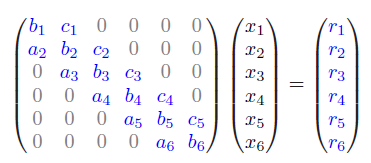这个一行最大个数为3个的对角矩阵，有一个公式为2*i+j-3,那么我们就要知道这个公式是怎么来的。
先看导出这种模式的代码
#include<bits/stdc++.h>
using namespace std;
int main(){

int b={0};//给b这个数组从1到200可以看检查我们的答案
for(int i=1;i<=200;i++){
b[i-1]=i;
}
for(int i=1;i<=6;i++){
for(int j=1;j<=6;j++){
if(abs(i-j)<=1){
cout<<b[2*i+j-3]<<" ";
}else{
cout<<"0 ";
}
}
cout<<endl;
}
}
编译效果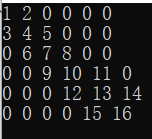如何导出公式
首先前面说了，对角矩阵的规律在其对角线中，那么我们先看这个对角矩阵总共有效的数字个数，从编译我们可以看出，一共16个，那么这16个有什么规律呢？从图片中我们不难看出，一行3个，一共6行，但是注意，第一行左半部分是没有数字的，并且最后对角线的又半部分也没有数字，那么一共的数字就是3*i-2，我们设定i为矩阵的行数；然后我们观察，找到对角线的数字个数，为1，4，7，10，13，16，我们拿出第三行看，7，当我们看这个7的时候，他的右边我们是不考虑的。所以，他右边也少一个数字，那么我们的对角线的规律也就是3*i-2；这个公式是对角线的数字，现在我们不仅要知道对角线的数字，我们还需要知道这个对角线左右两边的个数，那么我们不仅需要知道3*i-2我们还有知道3*i-2-1和3*i-2+1,现在我们的目的就是要给这3个公式合起来为一个式子，现在我们引用j。j为矩阵的列数，让j>=i-1且j<=i+1时打印j，而原来的3*i-2我们可以看成i+i+i+2,把其中的一个i用j代替，用j取3个数，i+1和i-1和i，这样我们就可以导出原有的公式2*i+j-2，那么为什么不是原始的公式2*i+j-3呢？是因为我们b是从b开始的，所以第一个数是b，所以要在2*i+j-2的基础上-1，我们就导出了公式2*i+j-3
从简单公式进阶
现在为一行最多3个，那么如果是5个应该怎么改这个公式呢？

#include<bits/stdc++.h>
using namespace std;
int main(){
int b={0};
for(int i=1;i<=200;i++){
b[i-1]=i;
}
for(int i=1;i<=6;i++){
for(int j=1;j<=6;j++){
if(abs(i-j)<=2&&i>1&&i<6){
cout<<b[4*i+j-6]<<" ";
}else{
if(abs(i-j)<=2&&i<=1){
cout<<b[4*i+j-5]<<" ";
}else if(abs(i-j)<=2&&i>=6){
cout<<b[4*i+j-7]<<" ";
}else{
cout<<"0 ";
}
}
}
cout<<endl;
}
cout<<endl;
}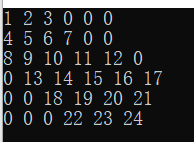首先在我们明白上面公式如何导出后，我们先导出对角线的数字，正常如果按照一行5个来算，一共的数字为5*i，然后我们看，每个对角线后存在2个我们不用的，就为5*i-2，但是第一行左边也有2个不用，就为5*i-4，现在分界点来了，在第二行，第二行中间数左半部分有部分没进入。所以我们以第一行为分界点，当i>1时，左边一共少2个，右边一共少2个，所以总共比正常的5i少5个就为5*i-4而从第一行以后到 i 行前就第二行少了一个，所以左边一个少3个，右边一共少2个，所以总共比正常少5个就为5*i-5。然后引入 j ，现在的j和之间有一点不同点就是要j>=i-2且j<=i+2 然后把其中的一个i改为j，两个公司就变为4*i+j-4和4*i+j-5同理,右边也有地方部分进入的，所以我们也要在右边做一个分界点，这个分界点就是6，那么和左边一样，当i==6时他也要在中间基础上少1,就是4*i+j-6最后从b开始上面两个公式各-1，公式变为4*i+j-5和4*i+j-6,4*i+j-7一行最多5个就结束了.
最终通用公式导出
当每行最多字符为7，矩阵边数为9时，编译运行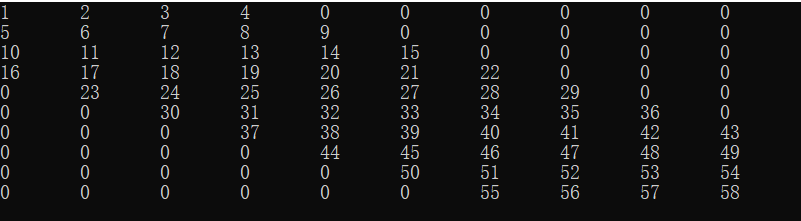现在我们把每行最多的个数设定为x，每次改变x最后的效果. 7. 还是重复之前的步骤，先找出总共正常的数字个数为x*i 8. 然后找右边缺少个个数,为**(x-1)/2** 9. 然后划分分界点，当最终的 i 左边有部分进入，最终的 i 和左边没有进入也就是下图3个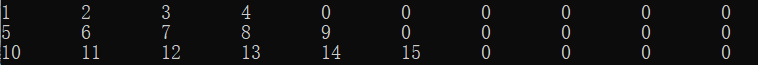和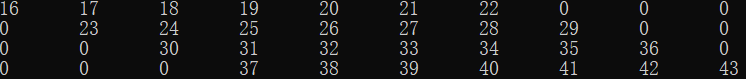和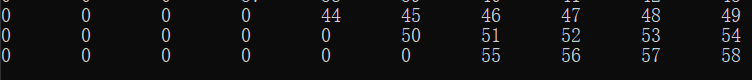先看中间的图的分界，他一共少了前面3+2+1个，那么他就是小了从1+···（x-1）/2，根据叠加，左边一共少了这些(x*x-1)/8，而右边少了(x-1)/2，所以中间部分的分界点的公式就是(x-1)*i+j-( x * x-1)/8-(x-1)/2-1, ( j 和-1)都和前面一样.接下来导出上变分界，上方叠加是从(x-1)/2-(i-1)+（x-1），所以根据叠加公式为,(i*(x-i))/2,说以上方的公式就是，(x-1)*i+j-(x-1)/2-(i * (x-i))/2-1，（j和-1和之前一样）最后看下半边界,左边缺少(xx-1)/8,而右边缺少从1+····(x-1)/2+i-10，根据叠加缺少((x-1)/2+i-10)((x-1)/2+i-11)/2,并且还缺少一个(x-1)/2，所以一共缺少(x-1) * i+j-((x-1)/2+i-10)*((x-1)/2+i-11)/2-(x * x-1)/8-(x-1)/2-1;
最后发一下写出的的代码
int x=7;
for(int i=1;i<=10;i++){
for(int j=1;j<=10;j++){
if(abs(i-j)<=(x-1)/2&&(x-1)/2-i<=0&&(x-1)/2+i<=10){
cout<<b[(x-1)*i+j-(x*x-1)/8-(x-1)/2-1]<<"\t";
}else{
if(abs(i-j)<=(x-1)/2&&(x-1)/2-i>0){
cout<<b[(x-1)*i+j-(x-1)/2-(i*(x-i))/2-1]<<"\t";
}else if(abs(i-j)<=(x-1)/2&&(x-1)/2+i>10){
cout<<b[(x-1)*i+j-((x-1)/2+i-10)*((x-1)/2+i-11)/2-(x*x-1)/8-(x-1)/2-1]<<"\t";
}else{
cout<<"0\t";
}

}
}
cout<<endl;
}
展开全文c++
• 压缩存储称矩阵存储时只需要存储上三角/下三角的数据，所以最多存储n(n+1)/2个数据（相当于1+2+…+n,即等差数列求和）。 对称矩阵压缩存储的对应关系：下三角存储i>=j, SymmetricMatrix[i][j] ==Array[i*(i+1)/2+j...
• 将整个图形的连接存储在邻接矩阵中。 用邻接链表来表示图之间的关系 在图中表示连接的最简单方法是在每个节点的数据结构中存储与其连接的节点的列表。该结构称为邻接列表。 例如，在航空公司图表中 每一个节点连接...
图的存储方式
在实践中，存储图最常见的策略是：
将每个节点的连接存储在邻接列表中。将整个图形的连接存储在邻接矩阵中。
用邻接链表来表示图之间的关系
在图中表示连接的最简单方法是在每个节点的数据结构中存储与其连接的节点的列表。该结构称为邻接列表。 例如，在航空公司图表中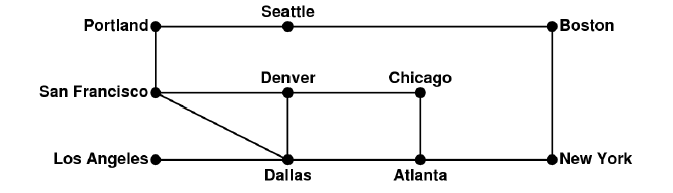每一个节点连接的相邻节点构成一个邻接表，就像这样：现在我们来讲将上图的内容抽象化： 假设我们现在有个有向图：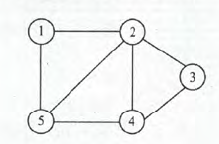根据定义，我们将每个节点的连接存储在邻接列表中。对于节点 1 2 3 4 5，有以下连接表（比如与节点1相连的节点有2和5，他们之间先后顺序可以是任意的）：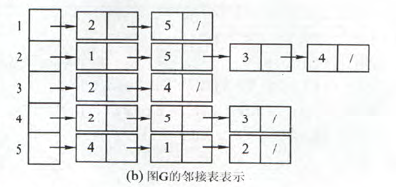当图为有向图的时候，按箭头的方向给出连接表即可：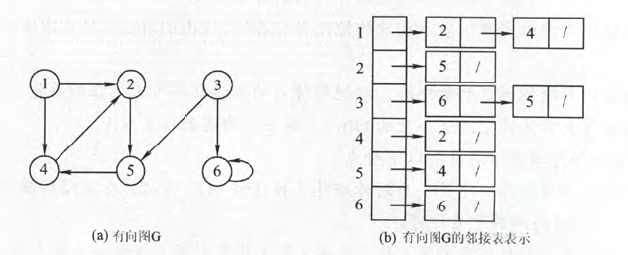使用邻接矩阵表示节点间的关系
虽然临接表提供了一种表示图形中连接的便捷方式，但是当操作需要搜索与节点关联的节点时，它的效率可能会低下。 例如，如果使用邻接列表表示，则确定是两个节点是否相互连接需要O（D）时间，其中D表示节点之间的度。 如果图中的节点都具有少量邻居，则搜索该列表的成本很小。 但是，如果图中的节点往往具有大量邻居，则此成本变得很高。 如果效率成为一个很关心的问题，那么我们可以通过在称为邻接矩阵的二维数组中表示节点之间的花费（即权重），该数组显示哪些节点已连接。 航空公司图的邻接矩阵如下所示：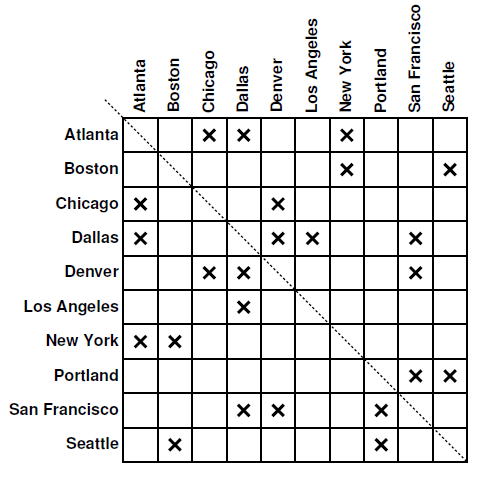通常空白部分我们用∝表示，意思是两个节点之间没有直接关联。 特别的，当图为无向图是，邻接矩阵是对称的（如上图所示），因为每个相邻节点之间有相互的关系。（这个时候，如果矩阵很大，可以采取压缩矩阵的方式将矩阵压缩。矩阵的压缩后面会提到） 一般的，如果无向图中两个节点相互连接，那么我们就记为1，否则记为0,。这个时候我们就可以得出只有0 1 的矩阵。 下面来加班一些具体的实例：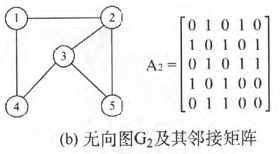上图中，第一行表示的是，对于节点1，它与自己本身不相连，记为0，与节点2相连记为1。与节点3不相连记为0，与节点4相连记为1，与节点5不相连记为0.由此得出矩阵的第一行应该是0 1 0 1 0.其他行以此类推。 对于有向图，可以按上述的逻辑进行推算：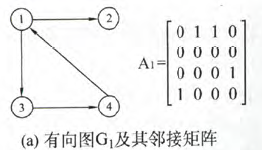还有一种图，叫做带权图。如下：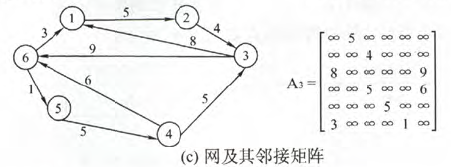所谓权，就是节点之间相互到达需要的花费。这时候我们的邻接矩阵记录的就是权的大小。当节点之间的距离需要逆向而行或者非直接连接的时候，这是我们表示距离不可达，即无穷。比如第一行，节点1到自己本身没有箭头可指，因此是无穷。而节点1到节点2需要花费5.而节点1到节点三不是直接相连的，也记为无穷。由此得出邻接矩阵每行的数据。
要使用邻接矩阵，必须将每个节点与其索引号相关联，该索引号指定该表中与该节点对应的列或行号。 作为图的具体结构的一部分，实现需要为图中的每个节点分配一行和一列的二维数组。 数组的元素是布尔值。 如果matrix [start] [finish]中的条目为真，则图中就有表示 start→finish的节点间的关系。
两种表示方式的空间复杂度
就执行时间而言，使用邻接矩阵比使用邻接列表快得多。 但另一方面，矩阵需要O（N^2）存储空间（如果不压缩），其中N是节点的数量。 对于大多数图形，邻接列表表示在空间方面往往更有效。 在邻接列表表示中，每个节点都有一个连接列表，在最坏的情况下，它的长度将是Dmax，其中Dmax是图中任一节点的最大度数，即从单个节点出发的最大连接数。 因此，邻接列表的空间成本是O（N×Dmax）。 如果大多数节点彼此连接，则Dmax将相对接近N，这意味着表示连接的成本对于两种方法是相近的的。 另一方面，如果图形包含许多节点但相对较少的互连（称为稀疏矩阵），则邻接列表表示可以节省相当大的空间。
除此之外，图的存储方式还有邻接多重表和十字链表法。
特点：
由上述可以看出，通常我们可以将边的类型定义为0或者1的枚举类型。无向图的邻接矩阵一定是对称矩阵（且唯一），对于规模较大的矩阵可以进行压缩。当图的边数较多的时候，适合采用邻接矩阵表示。反之当边数较少的时候适合采用邻接链表。
矩阵压缩
我们刚刚提到 ，对于规模较大的对称矩阵可以考虑进行压缩。那么什么是压缩呢？ 压缩存储，是指为多个值相同的元素只分配一个存储空间，并且对于0元素不分配空间。其目的就是要节省存储空间。我们通常可以对一些特殊的矩阵进行压缩。下面着重介绍三种（只考虑方阵）。
对称矩阵
定义：对于一个n阶方阵A[1…n][1…n],中的任意一个元素Aij，满足：
Aij = Aji //其中 1 <=1,且j < = n

那么就称为对称矩阵。通常可以将元素分成3大部分：上三角区，下三角区，对角线。：图中，A(n,1) = A(1,n), A(n,2) = A(2,n) 因此我们可以考虑直接用一维数组来存储一半的数据即可，另外一半的数据可以由之前的一半交换下标所得。假设我们新建一个数组B来存储：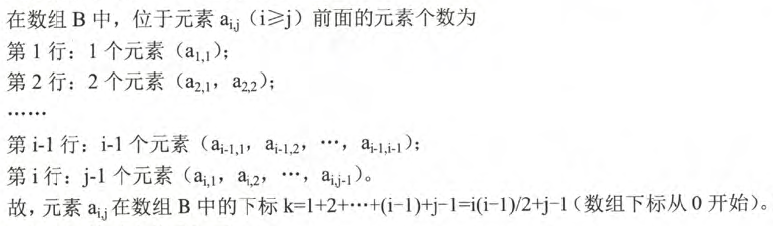其中:
1+2+3+4+....(i-1)   \\定位数组所在的行
（j -1）\\定位数组所在的列

因此得出如下关系（两个式子实际上是i，j 位置互换）：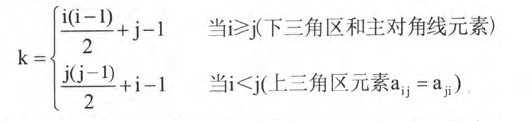三角矩阵
这种矩阵的特点是，上三角区的所有元素均为同一常量。其存储思想与对称矩阵类似。先存完下三角和主对角线的元素后，再存对角线上方的常量一次。
下三角矩阵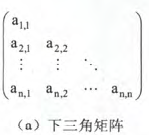对于下三角，存储方式完全与对称矩阵的下三角相同：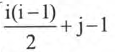对于下三角，由于存的都是常数，第一行有一个元素，第二行有两个元素，第三行有3个元素，那么第n行的n个元素（存常数），他所在的位置就是一个等差数列求和的过程。：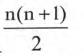因此其在内存中的存储为：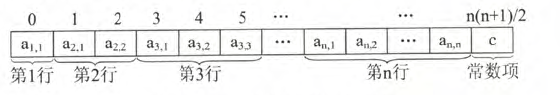也就说，常数项只占用一个存储位置。上三角矩阵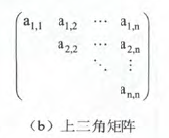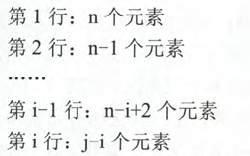按刚刚的方法可以很快得出位置关系：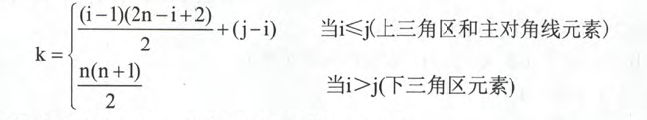从而得出存储图：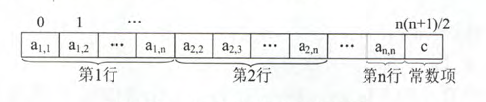三对角矩阵
对于n阶方阵A中的任一元素a（i，j），如果| i - j | >1（即行-列的绝对值大于1）的时候，有 a（i，j）= 0，那么矩阵A就被称为三对角矩阵。在三对角矩阵中，所有的主要元素都集中在以主对角线为中心的3条对角线中，其他区域的数值均为0.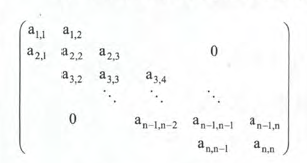采用压缩存储，其原理为，先将3条对角线上的元素，以按行优先方式存储在一维数组B中，且B0为a（1,1）。那么存储结构为：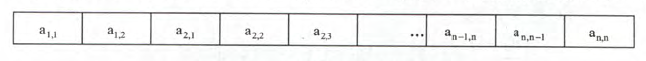因此，A中三条对角线的主元素A（i，j）的下标为：
k = 2i + j -3

反推，已知元素位置为k，那么其下标为：
i = [(k+1) /3 +1]   //中括号的意思是向下取整
j = k - 2i +3  //这里是求主元素下标公式的逆推

稀疏矩阵
这个很容易理解，就不用什么官方定义了，就是矩阵中，非零元素非常少的矩阵。（比如一个100 x 100的矩阵中，只有不到100个非零元素）。这种矩阵就是稀疏矩阵。 对于这种矩阵的存储也很好办，只要我们存储非零元素就好了。如果将行，列，值三者组成一个三元组，那么三元组的格式就是（行，列，值）。将其存在数组下标就很好办：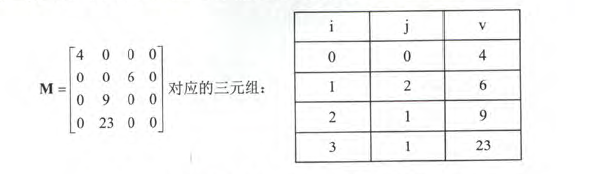注意，这里我们是指定了特定的地方存储，那么矩阵压缩存储后，就失去了随机存储的特性。
按行存储和按列存储
按行存储
这个也不看具体的公式定义，先上图：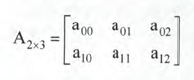上图是个二维数组，一个矩阵。按行存储，意思就是先把行从左往右读，每读到一个就存起来：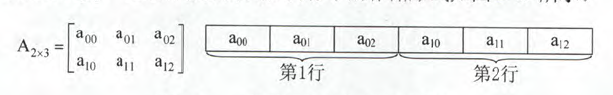这样就可以得出一个结论，元素a（i，j）所在的位置为：
i x (h +1) +j //h表示列的最大值，从0开始算
//i x (h +1) 可以定位到a（i，j）开始的位置a （i）
//再加上所在的列，可以计算出具体的位置。

用LOC（a（0，0））表示数组在内存中表示的物理位置，L表示每个元素的存储空间，那么a（i，j）的物理位置为（从0开始算）：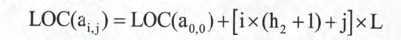按列存储
原理同上，放图自己分析：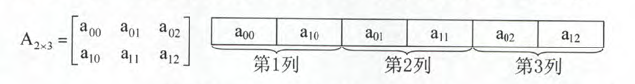展开全文存储方式
• 本篇文章主要介绍了C++实现稀疏矩阵压缩存储实例，小编觉得挺不错的，现在分享给大家，也给大家做个参考。一起跟随小编过来看看吧
• 矩阵压缩 由于稀疏矩阵中非零元素较少，零元素较多，因此可以采用只存储非零元素的方法来进行压缩存储。 由于非零元素分布没有任何规律，所以在进行压缩存储的时侯需要存储非零元素值的同时还要存储非零元素在数据结构 java
• 对称矩阵 n阶矩阵中任意一个元素aij都有aij=aji，则为对称矩阵 只存储主对角线+下三角区（按行优先存储在一维数组中） 数组大小：(1+n)*n/2 三角矩阵 三对角矩阵 稀疏矩阵 三元表（行，列，值） 十字链表...数据结构
• //矩阵压缩算法 //将矩阵压缩成另一个矩阵 //另一个矩阵第一行存储了之前矩阵的行数 列数 以及非0元素个数 //然后每一行都保存了非0元素的行数 列数 及元素值 public class Main { public static void main(String ...性能优化 算法
• ## 三角矩阵压缩

千次阅读 2018-03-16 13:10:32
三角矩阵压缩 关于三角矩阵的描述是，其非0元素呈三角状排列，三角矩阵又分上三角矩阵和下三角矩阵，如果我们用二维数组来储存三角矩阵的话，0元素会浪费很多的空间，因此我们可以用一维数组把矩阵进行压缩，下面给...
• 对称矩阵压缩实现原理c二维数组存储在c中矩阵的表示是用二维数组。那么首先要搞清楚数组行列与矩阵行列的对应。在c语言中二维数组是按行存储的。即顺序存储每一行。（第一行，第二行。。。最后一行） 看一下例子...二维数组
• 使用C语言，利用稀疏矩阵，完成矩阵压缩存储。详细可见博文：https://blog.csdn.net/qq_44075108/article/details/115435408
• ## csr_matrix矩阵压缩

千次阅读 2018-10-26 21:12:45
scipy库中的sparse.csr_matrix可对稀疏的np.array进行压缩处理，有两种方式：csr(Compressed Sparse Row marix) 和 csc(Compressed Sparse Column marix)。下面通过两个最常见的栗子说明一下。 scipy.sparse.csr_...csr_matrix
• 本篇文章以五子棋的棋局保存为背景，用c语言实现原始稀疏矩阵转换为压缩矩阵并且存储为文件，棋局复盘则读取文件将压缩矩阵转换为原始稀疏矩阵。 图解 假如有如下棋局，如果要保存，可以用1来表示红棋子，2来表示...c语言
• 数组（对称矩阵压缩存储） 设aij为n阶对称矩阵A中i行j列的数据元素，k为一维数组va的下标序号，则其数学映射关系为： 注意：数学中，n阶对称矩阵元素aij的下标满足条件：1≤i≤n和1≤j≤n 实践 具体代码如下...
• 写了一个pytorch框架下对LSTM的矩阵实现分块循环矩阵压缩的方法 参考这篇博客:https://blog.csdn.net/kuan__/article/details/116600433
• 1. 对称矩阵采用一维数组进行存储，例如test={ { 1 , 2 , 3 } , { 2 , 1 , 3 } , { 3 , 3 , 1 } }存进 ans [ ] 里面;for(int i=0;i&lt;3;i++)for(int j=0;j&lt;3;j++)ans[ len++ ]=test[ i ] [ j ];解...
• "压缩后：\n" ); for ( int i = 0 ; i (SIZE *( SIZE + 1 ) / 2 ); i++) printf ( " %d " , p1[i]); printf ( "\n" ); printf ( "解压前：\n" ); for ( int i = 0 ; i (SIZE *( SIZE + 1 ) / 2 )...数据结构 c++
• https://blog.csdn.net/swq463/article/details/85851718 对应的代码c
• 对于数组的压缩存储，一维数组主要使用哈夫曼压缩，多维数组主要采用矩阵压缩的形式，对特殊矩阵和系数矩阵进行压缩。 哈夫曼压缩 哈夫曼压缩是由哈夫曼树推广而来的，是哈夫曼编码的重要应用。哈夫曼树 ─ 即最优...哈夫曼
•技术
• matlab中squeeze函数用于删除矩阵中的维数为1的维（只适用于维数大于2的矩阵l,），比如执行下面的代码，随机产生一个1x2x3的矩阵A，然后squeeze（A）将返回一个2x3的矩阵，将第一维却掉(因为第一位大小为1)： ......